# Volume, Pan, Pi duration

Part of OrgMaker Notes

## Volume

I prepared an ORG file to play sounds at five equally spaced volume levels. That is, at 0, 1/4, 1/2, 3/4, and full volume levels. (To specify exact ORG volume levels, I first made an ORG file with the approximate volume levels I wanted, then I used a hex editor to get the volume levels to the exact values I wanted.) I played the test file and recorded the resulting sound into Audacity.

The ORG test volume levels recorded in Audacity.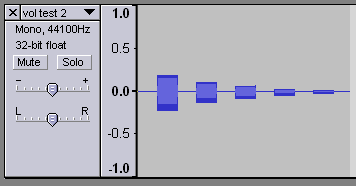I then examined the recordings in Audacity. I zoomed in on the waveform, scrolled the parts I wanted to measure so they were next to the amplitude ruler, and estimated the levels of the waveform to four decimal places. I noticed the center of the waveforms weren't centered on zero, so I measured the top and bottom point of each waveform and calculated the amplitude as half of the distance between the top and bottom. I then normalized the values so the maximum amplitude is 1.

Zooming in to measure the top, middle, and bottom points. (Click to enlarge.)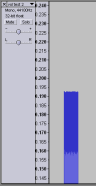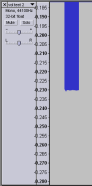When I tested the ORG volume levels, the most surprising result was an input level of zero was still quite audible. I found the following formula seems to fit.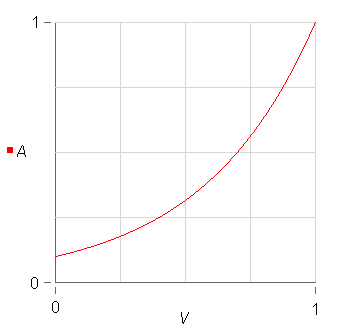V is relative ORG volume (0 to 1) A is relative amplitude (0 to 1) A = 10(V − 1)

## Pan

I examined the pan values the same way: I recorded ORG sounds at various pan values and examined the amplitudes in Audacity.

I noticed the ORG pan behaves like this:

• When the pan is centered, the left and right channel have the same amplitude.
• When the pan is left of center, the left channel's amplitude is the same as it was for centered pan and the right channel's amplitude is decreased.
• When the pan is right of center, the right channel's amplitude is the same as it was for centered pan and the left channel's amplitude is decreased.

Here are the formulas I found that fit.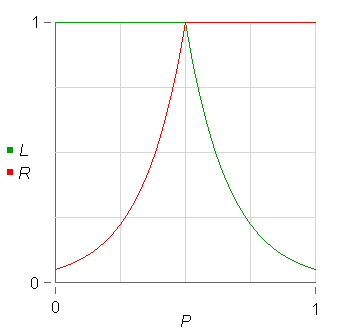P is relative ORG pan (0=left, 0.5=center, 1=right)
L is relative amplitude, left channel (0 to 1)
R is relative amplitude, right channel (0 to 1)

 L = { 1, 0 ≤ P ≤ 0.5 20(1 − 2P), 0.5 < P ≤ 1

 R = { 20(2P − 1), 0 ≤ P < 0.5 1, 0.5 ≤ P ≤ 1

## Pi duration

When I examined what recordings of melodic OrgMaker notes looked like in Audacity, I found that when the Pi checkbox is enabled, OrgMaker outputs a specific number of wave periods in each OrgMaker octave.

OrgMaker octaveperiods
04
18
212
316
420
524
628
732

In other words, octave 0 starts with 4 periods, each additional octave adds 4 periods.

Terminology reminder: In a periodic wave, the period is the length before the wave repeats. For example, if you use a sine wave, one period is one cycle of the sine wave.

Next: Pitch